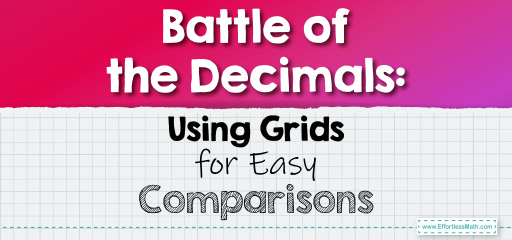# Battle of the Decimals: Using Grids for Easy Comparisons

Decimals can sometimes be tricky to compare, especially when they have different numbers of digits after the decimal point. One effective method to compare decimals is by using grids. Grids allow us to visually represent decimals, making it easier to see which one is greater or smaller. Let's dive into this method!## Comparing Decimals Using Grids

### Example 1:

Decimals to Compare: $$0.3$$ and $$0.25$$

Using a Grid:

Imagine a grid where each row represents a place value: tenths, hundredths, thousandths, and so on.

For $$0.3$$:

– Fill in 3 cells in the tenths row.

For $$0.25$$:

– Fill in 2 cells in the tenths row.

– Fill in 5 cells in the hundredths row.

By looking at the grid, it’s clear that $$0.3$$ fills more cells in the tenths row than $$0.25$$, making $$0.3$$ greater.

$$0.3 > 0.25$$

The Absolute Best Book for 5th Grade Students

### Example 2:

Decimals to Compare: $$0.47$$ and $$0.5$$

Using a Grid:

For $$0.47$$:

– Fill in 4 cells in the tenths row.

– Fill in 7 cells in the hundredths row.

For $$0.5$$:

– Fill in 5 cells in the tenths row.

By looking at the grid, even though $$0.47$$ has more filled cells overall, $$0.5$$ has more cells filled in the tenths row, making it greater.

$$0.5 > 0.47$$

Using grids to compare decimals offers a visual approach that can be especially helpful for those who are more visually inclined. It’s a great way to understand the relative sizes of decimals and can be a fun activity for students to practice!

### Practice Questions:

1. Which is greater: $$0.6$$ or $$0.58$$?

2. Compare $$0.72$$ and $$0.8$$. Which is larger?

3. Determine the larger decimal between $$0.345$$ and $$0.35$$.

4. Which is smaller: $$0.9$$ or $$0.89$$?

5. Compare $$0.123$$ and $$0.13$$. Which is smaller?

A Perfect Book for Grade 5 Math Word Problems!

1. $$0.6$$

2. $$0.8$$

3. $$0.35$$

4. $$0.89$$

5. $$0.123$$

The Best Math Books for Elementary Students

### What people say about "Battle of the Decimals: Using Grids for Easy Comparisons - Effortless Math: We Help Students Learn to LOVE Mathematics"?

No one replied yet.

X
51% OFF

Limited time only!

Save Over 51%

SAVE $15 It was$29.99 now it is \$14.99